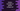# How to ceil all values of an array using python numpy ceil## Introduction :

Python numpy ceil method is used for ceiling all valeues of an array. It returns one ndarray or scalar. In this post, I will show you how to use it with one example.

### Syntax :

The syntax of numpy ceil is as like below :

``numpy.ceil(arr[,out])``

Here, arr : It is the input array_like data. out : It is an optional parameter. It can be a ndarray, None or tuple of ndarray and None. This is a location to put the result. It should be of the same shape as the inputs. If we don’t provide it or if it is None, this method returns one freshly allocated array.

### Example program :

Let me show you one example of the ceil method.

``````import numpy as np

array_one = np.array([1, 2.4, 2.5, 2.6, 2.9, -2.4, -2.5])
array_two = np.array([(4.4, 4.5), (5.1, 5.9), (1.1, 1.9)])

print('{} : {}'.format(array_one, np.ceil(array_one)))
print('{} : {}'.format(array_two, np.ceil(array_two)))

array_three = np.array([1, 4.4, 5.5, 7.6, -2.9, -12.4, -29.5])
array_cp = np.zeros(7)
np.ceil(array_three, out=array_cp)
print('{} : {}'.format(array_three, array_cp))``````

Here, we are using ceil with three arrays. array_one is a 1D array, array_two is a 2D array. The first two print statements printed the ceil values for these arrays. array_three is a 1D array and the last ceil method copies the result to the array array_cp, which is an array initialized with zeroes.

It will print the below output :

``````[ 1.   2.4  2.5  2.6  2.9 -2.4 -2.5] : [ 1.  3.  3.  3.  3. -2. -2.]
[[4.4 4.5]
[5.1 5.9]
[1.1 1.9]] : [[5. 5.]
[6. 6.]
[2. 2.]]
[  1.    4.4   5.5   7.6  -2.9 -12.4 -29.5] : [  1.   5.   6.   8.  -2. -12. -29.]``````

As you can see here that the values are ceiled in all arrays. The third print statement prints the value of array_three and array_cp. The result is copied to array_cp.